## Line to Line Fault (LL):

Figure 11.7 shows a Line to Line Fault at F in a power system on phases b and c through a fault impedance Zf. The phases can always be relabelled, such that the fault is on phases b and c.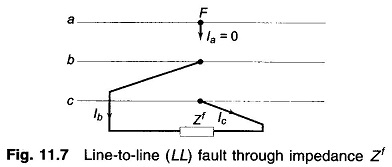The currents and voltages at the fault can be expressed as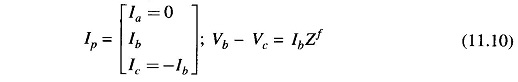The symmetrical components of the fault currents are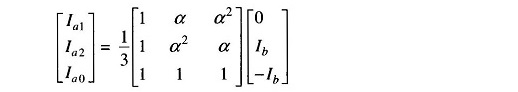from which we get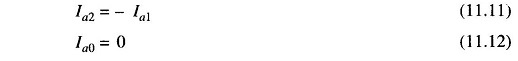The symmetrical components of voltages at F under fault are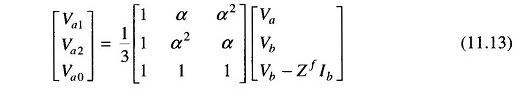Writing the first two equations, we have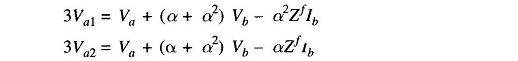From which we get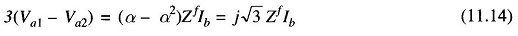Now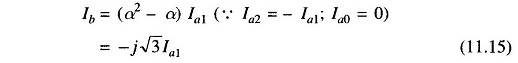Substituting Ib from Eq. (11.15) in Eq. (11.14), we get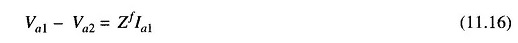Equations (11.11) and (11.16) suggest parallel connection of positive and negative sequence networks through a series impedance Zf as shown in Figs. 11.8a and b. Since Ia0 = 0 as per Eq. (11.12), the zero sequence network is unconnected.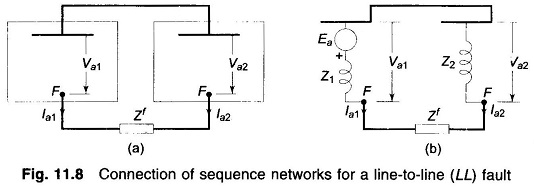In terms of the Thvenin equivalents, we get from Fig. 11.8b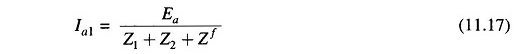From Eq. (11.15), we get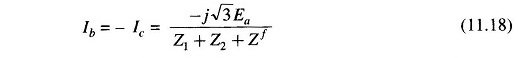Knowing Ia1,we can calculate Va1 and Va2 from which voltages at the fault can be found.

If the fault occurs from loaded conditions, the positive sequence network can be modified on the lines.

Scroll to Top